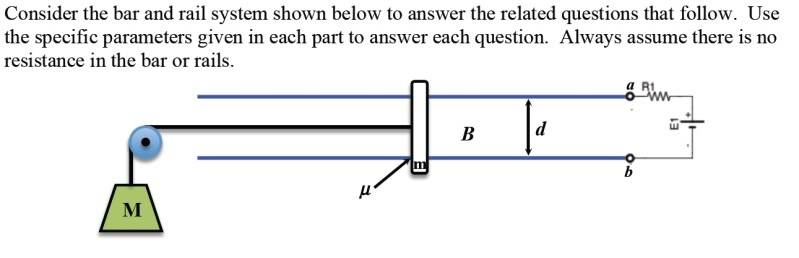# Motional emf

## Homework Statement

Here is the picture:I have an upcoming test, and I cannot seem to get any questions like this one. Help is greatly appreciated.

I calculated the net force, such that Fnet=ma. There exists a magnetic force due to the current in the wire. The mass m is negligible, ie 0kg. Also, assume the only resistence is R1 and E=36V. M=2kg, and B is directed into the page at 0.7T. Also, d=0.8m. Thus,

Fb-Mg=ma
ILB-Mg=ma
(36A)(0.8m)(0.7T)-(2kg)(9.81N/kg)=ma
0.54N=ma

Hence, there is an acceleration to the right.

From here I go absolutely blank. I have spent a lot of hours on these types of questions but I cannot comprehend them. Can someone please give me a hand.